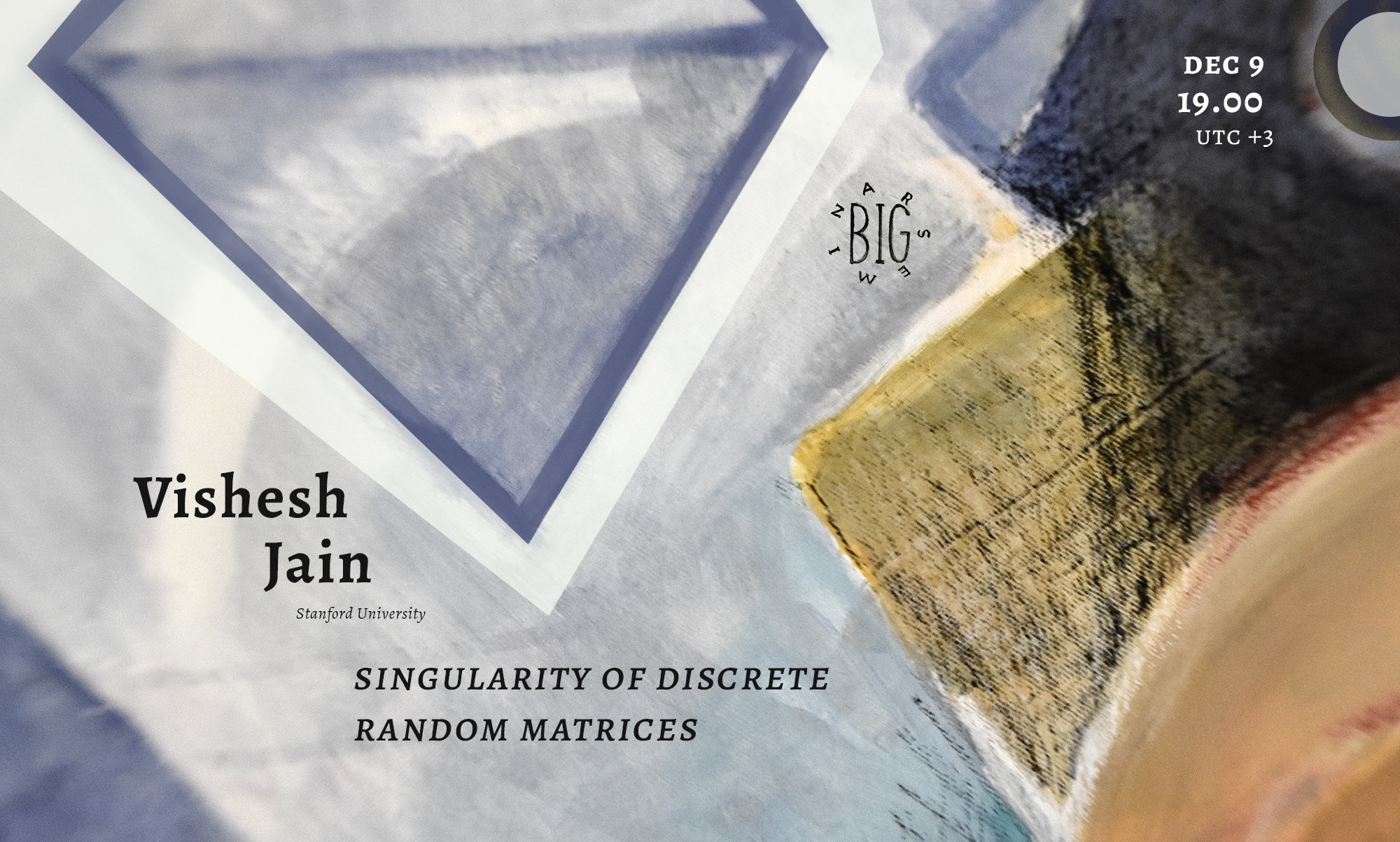# Vishesh Jain “Singularity of discrete random matrices” | Big SeminarDecember 9, 2021
19.00 MSK (UTC +3)
Talk on Big Seminar

# Vishesh Jain "Singularity of discrete random matrices"

Vishesh Jain from Stanford University will give the talk "Singularity of discrete random matrices" on the labs' Big Seminar.

The talk will be held in zoom
Password: first 6 decimal places of $\pi$ after the decimal point
Let $M_n$ be an $n\times n$ random matrix whose entries are i.i.d copies of a discrete random variable $\xi$. It has been conjectured that the dominant reason for the singularity of $M_n$ is the event that a row or column of $M_n$ is zero, or that two rows or columns of $M_n$ coincide (up to a sign). I will discuss joint work with Ashwin Sah (MIT) and Mehtaab Sawhney (MIT), towards the resolution of this conjecture.